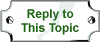Welcome to the Utopia Forums! Register a new account
The current time is Sun Oct 25 20:24:32 2020

Utopia Talk / General Talk / Pi!
 McKobb Member Sat Nov 17 03:14:57 Yup tumbleweed the wanderer Tue Nov 20 00:50:07 Fifty is the smallest number that is the sum of two non-zero square numbers in two distinct ways: 50 = 12 + 72 = 52 + 52. It is also the sum of three squares, 50 = 32 + 42 + 52, and the sum of four squares, 50 = 62 + 32 + 22 + 12. It is a Harshad number. There is no solution to the equation φ(x) = 50, making 50 a nontotient. Nor is there a solution to the equation x − φ(x) = 50, making 50 a noncototient. tumbleweed the wanderer Tue Nov 20 00:51:32 ...some of those 2's are exponents Cherub Cow Member Tue Nov 20 00:57:57 "...some of those 2's are exponents" Oh, good! I was seriously confused and wondering if I was looking at some upper-level math where normal rules do not apply :D -=-=- Fixing: 50 = 1² + 7² = 5² + 5². It is also the sum of three squares, 50 = 3² + 4² + 5², and the sum of four squares, 50 = 6² + 3² + 2² + 1². McKobb Member Tue Nov 20 01:34:28 At first I thought your talk of nontotients was just odd! tumbleweed the wanderer Tue Nov 20 11:41:01 nontotients are a good Thanksgiving topic to avoid politics or how there's a tremendous amount of seemingly pointless math McKobb Member Tue Nov 20 16:11:36 Maths are for childfen! Agri Patron Tue Dec 25 05:36:26 "Oh, good! I was seriously confused and wondering if I was looking at some upper-level math where normal rules do not apply :D " Same here! tumbleweed the wanderer Tue Dec 25 11:31:18 it was a copy/paste, blame Al Gore show deleted posts

 Your Name:Your Password: Your Message: